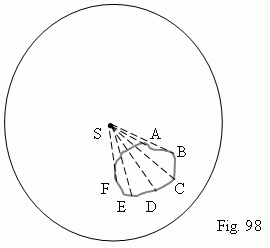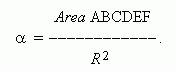# Solid angles

Solid angle. Measure of a solid angle. Steradian.

A solid angle is a part of a space, concluded inside of one part of a conic surface with a closed directrix. A solid angle is measured by a piece of spherical surface  ( ABCDEF,  Fig.98 ).A measure of a solid angle is a ratio of an area ABCDEF to a square of radius of the ball:A measure unit of a solid angle is a steradian – a solid angle, cutting out a spherical surface an area, equal to an area of square, a side of which is equal to radius. This measure of solid angles is similar to the radian measure of plane angles.